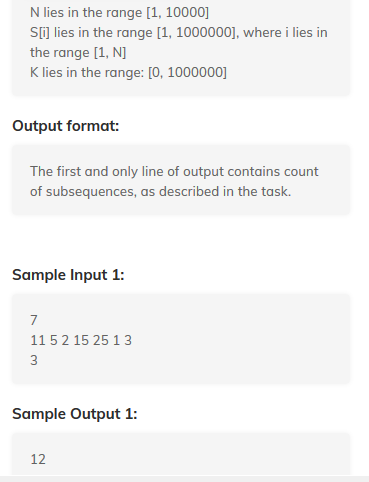# Can we improve worst time complexity of this question? [Solved]

Given an array, we have to calculate how many subarrays whose absolute difference of first and last element is less than or equal to K.

My approach :

``````    lli n;
cin>>n;
lli a[n];
scanarr(a,n);
lli k;
cin>>k;
sort(a,a+n);
lli count = 0;
for(lli low = 0, high = 0 ; high<n;high++)
{
for(;abs(a[high]-a[low])>k ; low++);

count += (high-low+1);
}

print(count);
``````

This code is optimized but in worst case it is O(N2)

similar question: “https://www.geeksforgeeks.org/pairs-difference-less-k/

This question is also asked in CN contest, I thought this approach will not work as Array size is long but it works.Help @galencolin @carre @aneee004

As S_i < 10^5, I think we can use a BIT?

I think this should work:

Code
``````BIT bit(MAXA);
for(int i = 0; i < n; i++) {
ans += bit.get(max(0, A[i] - k), min(A[i] + k, MAXA));
}
``````

[EDIT] The code is for \le k, if you want < k, then modify the query range appropriately.

4 Likes

I am confused if sorting will work. But My approach would be contribution towards the sum.

Let’s say we need to find sub arrays which end at `i`, then this means first element of the subarray must be in range (a[i]-k,a[i]+k)

So you just need to add frequency of these range sum, for that you can maintain a frequency array and find sum in O(log(n)) using segment tree or BIT.

So overall complexity : O(nlog(n))

Algorithm:

``````for i in range [1,n]:
ans += range_sum_query(max(0,a[i]-k+1),min(a[i]+k-1, MAX_LIMIT))
update(a[i],1)
``````
2 Likes

Can we do like that ( tell me if I m wrong ) , after sorting for each element by applying Binary search we will find the element X such that abs(X-current element) <=K ,Like this-

So overall complexity is O(nlogn + nlogn )

If you are sorting, how will you find subarrays, order must be maintained to find subarrays.

Bro we have to count all subarrays whose first and last element difference is less than or equal to K that means simple count all pairs whose abs difference is less than K

3 Likes

Shit yeah, this approach makes me feel dumb. I over-engineered a simple solution.

1 Like

Lmao , So can I use the approach which I mentioned later ?

Makes sense actually, I am looking if there is issue in code. Approach seems fine.

I think this will work fine

``````sort(all(arr))
long long ans = 0
for(int i = 0; i < n; ++i){
int index = upper_bound(all(arr),a[i]+k-1) - arr.begin();
ans += index - i - 1;
}
``````

I think1 Like

Yeah HashMap is enough i guess.

``````Sort array
for  i = 0 to n :
cnt += (i-max_index_from_0_to_i_such_that(abs(a[i] - a[index]) <=k)) +1
``````

How?

Wait I will submit and tell u if it is right or wrong.

Yes this one seems right too.

1 Like

Sorting is predetermining the complexity bound of O(N log(n)).

Suppose if a desired sub-array ends at i. Then we only have to look for no. of times a[i]+k and a[i]-k occurs before i. If the numbers are in range we can create a frequency array, else we can use a HashMap. Either way complexity will be O(n).

EDIT 1: I didn’t read the part less than equal to. Sorry MY bad

1 Like

But how do you query a frequency array range sum in O(1)?
It’s not diff = k, it’s diff < k.

[EDIT]. Lol. Alright.

2 Likes

WA bro

``````7
11 5 3 2 25 50 1
3

``````

Please tell me we just didn’t discuss solution to a live test